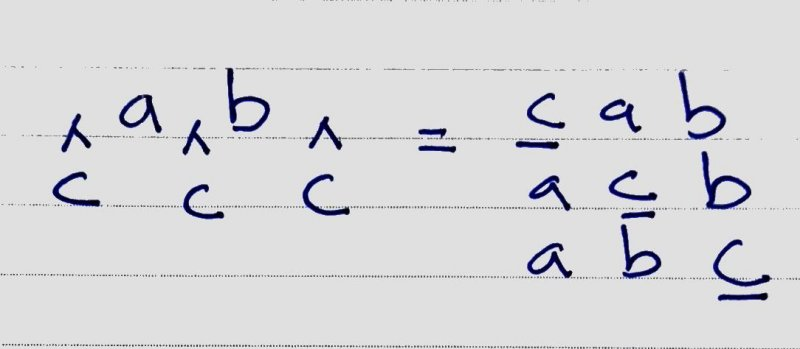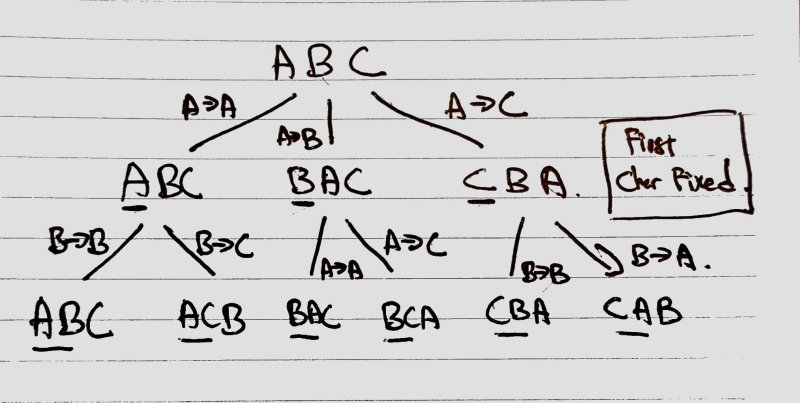# Generate All Permutations of String without Duplicates (Python, Recursion)

February 21, 2019
Generate all combinations

Expected output

``````'abc' = ['abc', 'bac', 'bca', 'acb', 'cab', 'cba']
``````

## Solution 1

Try a few input and output samples manually.

``````P('a') = ['a']
P('ab') = ['ab', 'ba']
P('abc') = ['cab', 'acb', 'abc', 'cba', 'bca', 'bac']
``````

Notice that to generate `P('abc')`, we take the output of `P('ab') = ['ab', 'ba']` and try to append `'c'` at each index/position `'ab'` (begin, middle, end).It seems like if we know the previous output `P(n-1)`, we can generate the current output `P(n)`. This sounds like a recursive solution.

``````def generate_permutations(text):
# validation
if text is None:
return None

# base, this is required as seeding value
if len(text) == 0:
return [""] # one empty element is required ensure the words loop run once

# else, we can seed with the following as well
'''
# if len(text) == 0: # base 0
#    return []
if len(text) == 1: # base 1
return [text]
'''

results = []
first = text
remainder = text[1:]

words = generate_permutations(remainder)
# print(first, remainder, words)
for word in words:
for i in range(len(word)+1):
# insert first char at each index/position of word
s = word[:i] + first + word[i:]
results.append(s)

return results
``````

## Solution 2

``````P(a,b) = ab, ba
P(b,c) = bc, cb
P(a,c) = ac, ca
``````

To generate `P(a,b,c)`

``````{ a +P(b,c) }  + { b + P(a,c) }  + { c + P(a,b) }
``````

Observe that we need to try `each character` + `P(remaining character)`.

``````def generate_permutations(text):
size = len(text)

results = []

# seed
if size == 0:
results.append("")
return results
# or
'''
# if size == 0:
#    return []
if size == 1:
return [text]
'''

for i in range(size): # loop each character
# generate str without current character (remaining character)
remainder = text[0:i] + text[i+1:]

# if text[i] = 'a', remainder = 'bc'
partials = generate_permutations(remainder)

# append current char to P(remaining character)
for s in partials:
results.append(text[i] + s)

return results
``````

Big O Time:

How many time generate_permutation is called.

`partials = generate_permutations(remainder)`

For P(‘abc’), it is 3 * 2 * 1 = 3!, so it is `O(n!)` (since there is no caching/memoization, and `n = n-1 = n`)

`for i in range(size)`

We need to loop through each char in ‘abc’, so it is `O(n * n!)`.

Based on Cracking the Coding Interview: 189 Programming Questions and Solutions by Gayle Laakmann McDowell.

Since we are calling permutation 0(n * n!) times (as an upper bound), and each one takes 0(n) time, the total runtime will not exceed O (n^2 * n!).

PS: It seems like I got `O(n * n!)` right, but the author also take into account string concatenation `remainder = text[0:i] + text[i+1:]` and print/append of result `results.append(text[i] + s)`, which require `O(n)` in each node.

## Solution 3

Observer the following diagram``````Level 0:
- Iterate a, b, c
- Swap char[first] with char[first], char[second], char[third]: a->a, a->b, a->c
- Fist character is done/fixed

Level 1:
- Iterate: it could be abc, bac or cba
- First character is done/fixed
- Swap char[second] with char[second], char[third]

Continue
``````
``````def generate_permutation(text):
# convert string to list of char to allow swapping
_generate_permutation(list(text), 0, len(text)-1)

def _generate_permutation(text, start, end):
# base
if start == end:
print(''.join(text))
return

for i in range(start, end+1):
text[start], text[i] = text[i], text[start]
_generate_permutation(text, start+1, end)
# backtrack like a tree, to restore previous state
text[start], text[i] = text[i], text[start]
``````